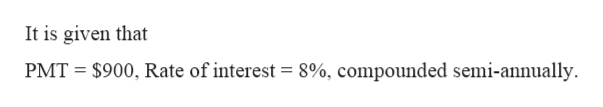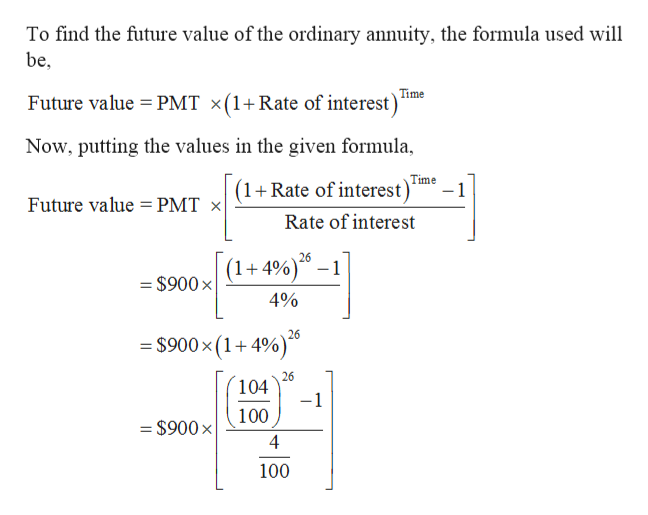# Find the future value of the ordinary annuity. Interest is compounded annually unless otherwise indicated.PMT=\$900, 8% interest compounded semiannually for 13 years.

Question
124 views

Find the future value of the ordinary annuity. Interest is compounded annually unless otherwise indicated.

PMT=\$900, 8% interest compounded semiannually for 13 years.

check_circle

Step 1

Given: -help_outlineImage TranscriptioncloseIt is given that PMT = \$900, Rate of interest = 8%, compounded semi-annually. fullscreen
Step 2

To find: -

Step 3

Calculation...help_outlineImage TranscriptioncloseTo find the future value of the ordinary annuity, the formula used will be, Time Future value = PMT ×(1+Rate of interest)* Now, putting the values in the given formula, Time -1 (1+Rate of interest) Future value = PMT × Rate of interest 26 (1+4%)" – 1 = \$900 x 4% 26 = \$900x (1+4%)ª 26 104 -1 100 = \$900× %3D 4 100 fullscreen

### Want to see the full answer?

See Solution

#### Want to see this answer and more?

Solutions are written by subject experts who are available 24/7. Questions are typically answered within 1 hour.*

See Solution
*Response times may vary by subject and question.
Tagged in
MathAlgebra

### Other